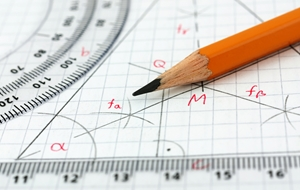What to expect of junior high math
MONDAY, OCTOBER 27, 2014 10:50 AMJunior high is a transitional time for students. Not only are they socially transitioning, they are also gaining the knowledge they need to prepare them for complex topics they'll learn in high school. Under the Common Core State Standards, students build foundational math knowledge in elementary school, then move on to important skills that will help them get ready for algebra, geometry and perhaps even calculus. If you're curious what junior high math is like with Common Core-aligned curricula, here's a closer look at the Standards' benchmarks for those grades:

Grade 6 of Common Core math focuses on five key areas: ratios and proportional relationships, expressions and equations, the number system, statistics and probability, and geometry. Each of these subject areas build off the knowledge students already have, such as multiplication, division and fractions. They tend to work with only one variable in algebraic problems, and use formulas for surface area and volume in geometry. In essence, students in grade 6 take the skills they already have in math and apply them in a more refined way.

Example problem: Here is an example of a problem students may solve in the expressions-and-equations category:

Write the following expression in an algebraic equation: A number, x, decreased by the sum of 2x and 5.

The answer is x-(2x+5). Students must understand what "sum," "decreased" and "and" mean in math terms. Sum is what one gets from adding. Decreased refers to subtraction, and "and" refers to adding. These definitions are things students learned in early grades and can now apply to algebra and other situations.

Math topic areas for grade 7 are the same as grade 6. The major difference is that students apply most of the concepts to real life. At this point, they have a good understanding of addition, subtraction, multiplication and division, and can use those basic principles to express problems in a variety of ways. They may have to create an algebraic expression based on some information, apply geometry formulas to a real-world situation or determine chance in statistics. Furthermore, students now use fractions in equations.

Example problem:

A kitchen store is having a sale on all dining sets for 70 percent off the original price. Tom has a coupon for an additional 20 percent off the discounted price. If the plate set Tom wants is originally \$40, how much will he pay with both discounts before tax?

The answer is \$9.60. The sale will take \$28 off the original \$40, leaving a price of \$12. Tom's coupon removes another \$2.40 from the set, making the final cost \$9.60 before tax.

Grade 8 is broken down to include functions, the number system, geometry, statistics and probability, and expressions and equations. Students are introduced to complex math concepts, such as irrational numbers, systems of equations, linear equations and the Pythagorean Theorem. Grade 8 prepares students for high school math, which is broken down by subject. The content paves the way for algebra and geometry understanding by helping students really grasp the core fundamentals of each topic.

Example problem:

Solve for x. x=16. What is x?

This problem gets into squares. In this example, the answer is 4, as 4 times itself (or 4 two times) is 16. Eventually, students will take this knowledge and search for the square root of number that does not  have a perfect square. This involves decimals.

Each grade level of Common Core math lays down the foundation for the next year's content. As such, junior high is an important time. Students gain the knowledge they'll need when tackling the challenging work of high school.RELATED ARTICLES All About TerraNova 3 Testing Teaching gifted kids in normal classes Helping your kids continue learning this summer More..
 NEWS CATEGORIES NEWS ARCHIVE